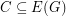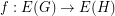Importance: High ✭✭✭
 Author(s): Jaeger, Francois
 Subject: Graph Theory » Coloring » » Edge coloring
 Keywords: cubic edge-coloring Petersen graph
Conjecture   Letbe a cubic graph with no bridge. Then there is a coloring of the edges ofusing the edges of the Petersen graph so that any three mutually adjacent edges ofmap to three mutually adjancent edges in the Petersen graph.
Ifis a graph andwe say thatis a binary cycle if every vertex in the graphhas even degree. Ifis a graph andis a map, we say thatis cycle-continuous if the pre-image of every binary cycle is a binary cycle. The following conjecture is an equivalent reformulation of the Petersen coloring conjecture.